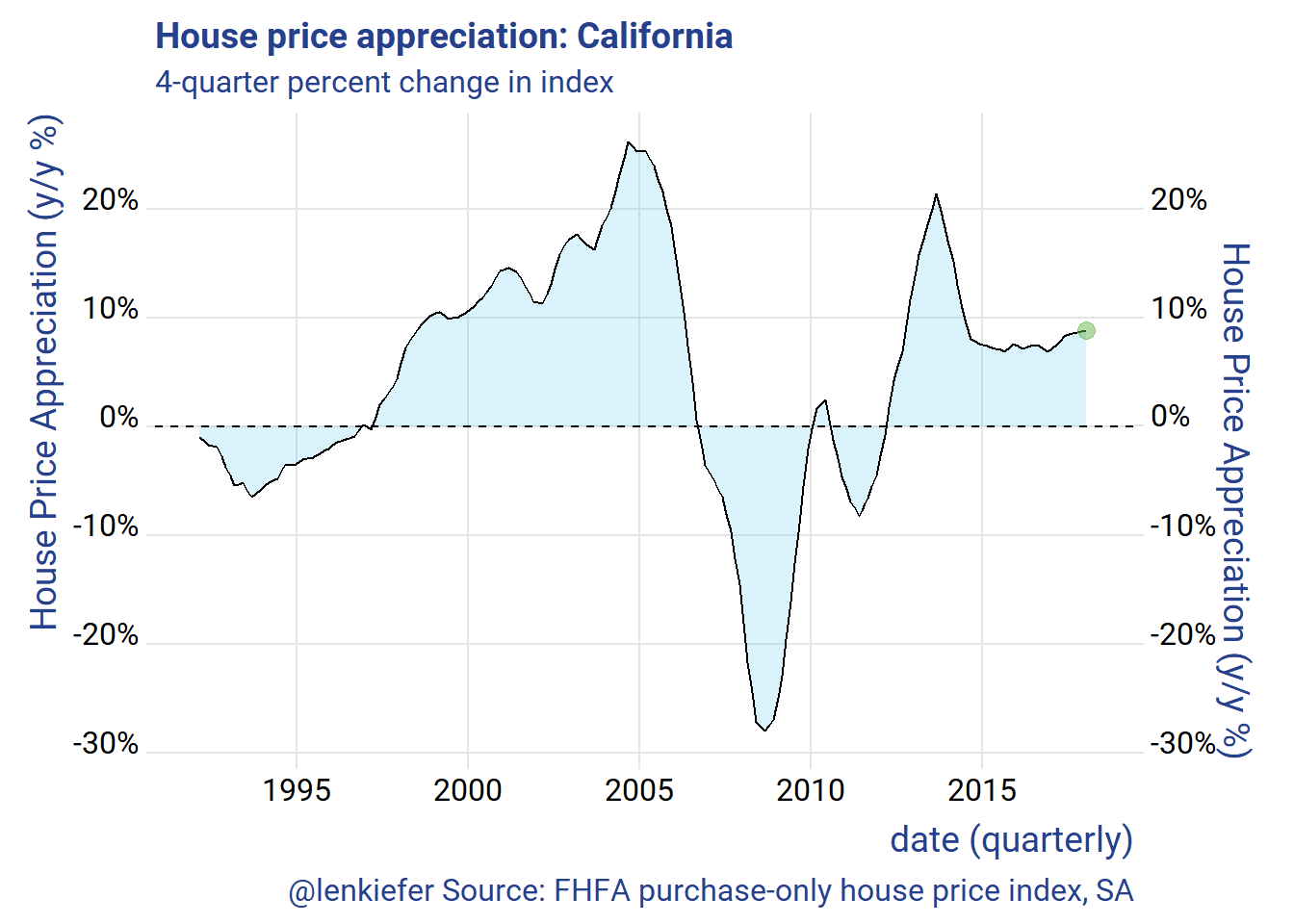# Housing in the Golden State

## I am headed out west, to California to talk housing at the Western Secondary Market Conference. Like many western states, California is facing a imbalance between housing supply and housing demand. Strong economic growth has bolstered demand, but supply has not kept up. The result is an acceleration in house prices.

I am headed out west, to California to talk housing at the Western Secondary Market Conference. After my talk they might post my slides online somewhere. If they do I’ll link to them, but for now you can get a preview in this twitter thread.

Like many western states, California is facing a imbalance between housing supply and housing demand. Strong economic growth has bolstered demand, but supply has not kept up. The result is an acceleration in house prices.

In this post, let’s examine some recent trends in the California house prices.

Per usual I’ll make charts with R and you can too with the code.

suppressMessages(
{
library(tidyquant)
library(tidyverse)
library(lubridate)
library(data.table)
library(ggridges)
library(extrafont)
}
)

Get some data:

suppressMessages(  {

# Get house price data ----

df.hpi <- mutate(df.hpi,date=as.Date(ISOdate(yr,period*3,1)))

df.hpi <- df.hpi %>% group_by(level,place_name) %>% mutate(hpa=index_sa/lag(index_sa)-1,
hpa4 = index_sa/lag(index_sa,4)-1) %>% ungroup()

# list of CA index
# use grepl("CA",unique(df.hpi$place_name)) to find California df.ca <- filter(df.hpi,grepl("CA",place_name)| place_name=="California" , hpi_type=="traditional", hpi_flavor=="purchase-only") df.us <- filter(df.hpi, place_name=="United States", hpi_type=="traditional",hpi_flavor=="purchase-only", frequency=="quarterly") }) # Make Animations Let’s make an animated line plot. First, let’s make a line plot. The code below generates a static plot. library(animation) library(tweenr) dfp <- filter(df.ca,level=="State",year(date)>1991) %>% select(date,hpa4) %>% mutate(day=1+(row_number()-1)*7, ease="linear", rate_label=as.factor(as.character(round(hpa4,0)))) plot_data_tween<-tween_elements(dfp, time = "day", group="ease", ease="ease", nframes = nrow(dfp)*2) df_tween_appear <- tween_appear(plot_data_tween, time='day', nframes = nrow(dfp)*2) make_plot_appear <- function(i){ p <- ggplot(data=df_tween_appear, aes(x=date,y=hpa4))+ geom_area(data= .%>% filter(.frame==i, .age> -3.5), color=NA, fill=rgb(9, 177,240, maxColorValue = 256),alpha=0.15 ) + geom_line(alpha=0)+ geom_hline(yintercept=0, linetype=2)+ geom_line(data= .%>% filter(.frame==i, .age> -3.5) ) + geom_point(data= .%>% filter(.frame==i, .age> -3.5) %>% tail(1), color=rgb(103,180,75, maxColorValue = 256), size=3, alpha=0.5)+ theme_ridges(font_family="Roboto")+ scale_y_continuous(labels=scales::percent, sec.axis=dup_axis())+ theme(text = element_text(color = "#27408b"))+ labs(y="House Price Appreciation (y/y %)",x="date (quarterly)", title="House price appreciation: California", subtitle="4-quarter percent change in index", caption="@lenkiefer Source: FHFA purchase-only house price index, SA") return(p) } make_plot_appear(max(df_tween_appear$.frame))Then we can use the animation packaged to animate.

oopt<-ani.options(interval=1/20)
saveGIF({for (i in 1:max(df_tween_appear$.frame)){ g<- make_plot_appear(i) + geom_hline(yintercept=0, linetype=2) print(g) print(paste(i,"out of",max(df_tween_appear$.frame)))
ani.pause()
}
for (ii in 1:2){
print(g)
ani.pause()
}

}, movie.name = "YOURDIRECTOR/hpa.gif", ani.width=700, ani.height=600,scale=1.2)  #set YOURDIRECTORY to where you want to save the file